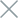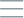• Home
• Tags
• Series
•# Local Search Algorithm in PHP: Understanding, Example & Implementation

The Local Search Algorithm is a significant approach in PHP programming, utilized to find the best solution within a limited search space. This algorithm is commonly applied in optimization problems, searching for optimal configurations, and addressing optimization challenges.

## How the Local Search Algorithm Works

The Local Search Algorithm focuses on improving an existing solution through small steps. It involves the following steps:

1. Identify Initial Solution: The algorithm starts with an initial solution for the problem.
2. Define Neighborhood Space: The algorithm defines the neighborhood space of the current solution, which includes solutions that can be obtained by making minor changes.
3. Evaluate Neighbor Solutions: The algorithm evaluates the quality of neighbor solutions by comparing them to the current solution.
4. Select Better Solution: If a neighbor solution is better than the current solution, the algorithm chooses the neighbor solution as the current solution. This process is repeated until no further improvements are possible.

• Effective for Large Search Spaces: The local search algorithm is often efficient with larger search spaces compared to global search algorithms.
• Ease of Implementation: This algorithm is generally easy to implement and can be customized for specific problems.

• Lack of Global Search Guarantee: This algorithm may lead to the best local solution that is not the globally optimal solution.
• Initialization Dependency: The algorithm's results can be influenced by the initial solution.

## Example and Explanation

Consider a simple optimization problem: finding the smallest value of the function \$f(x) = x^2\$ within the range from -10 to 10 using the Local Search Algorithm in PHP.

``````function localSearch(\$function, \$initialSolution, \$neighborhood, \$iterations) {
// Implementation of local search algorithm
// ...
}

\$function = function(\$x) {
return \$x * \$x;
};

\$initialSolution = 5;
\$neighborhood = 0.1;
\$iterations = 100;

\$optimalSolution = localSearch(\$function, \$initialSolution, \$neighborhood, \$iterations);
echo "Optimal solution: \$optimalSolution";
``````

In this example, we use the Local Search Algorithm to find the smallest value of the function \$f(x) = x^2\$ within the range from -10 to 10. The algorithm searches for neighboring solutions by making small changes to the value of \$x\$. After each step, the algorithm selects a better neighbor solution as the current solution. The result is a value of \$x\$ close to the minimum value of the function \$f(x)\$ within the specified range.

While this example illustrates how the Local Search Algorithm can optimize a value within a limited scope, it can also be applied to other optimization problems in PHP, such as finding optimal parameters for a model or optimizing system configurations.